• ①学习多变量系统状态空间表达式的建立方法、了解统状态空间表达式与传递函数相互 转换的方法； ②通过设计编程、上机调试，掌握多变量系统状态空间表达式与传递函数相互转换方法。
• G(s)=N(s)D(s)G(s)=\frac{N(s)}{D(s)}G(s)=D(s)N(s)​ 的串联分解形式 可控标准型 可观测标准型      实例 G(s)=N(s)D(s)G(s)=\frac{N(s)}{D(s)}G(s)=D(s)N(s)...状态空间表达式 其中：    ...


G

(

s

)

=

N

(

s

)

D

(

s

)

G(s)=\frac{N(s)}{D(s)}

的串联分解形式
可控标准型可观测标准型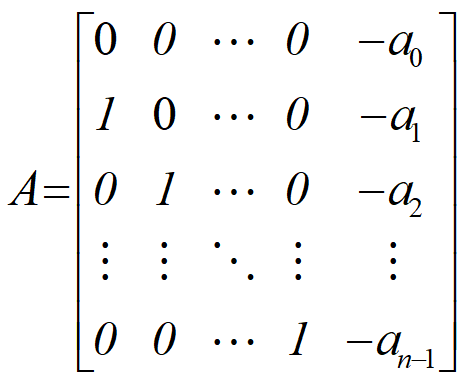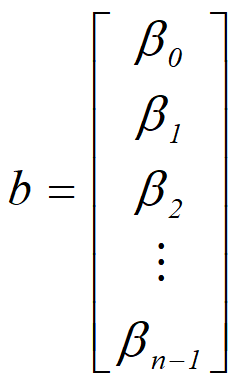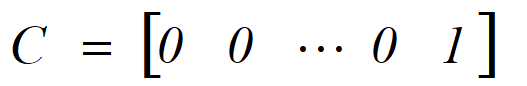实例G

(

s

)

=

N

(

s

)

D

(

s

)

G(s)=\frac{N(s)}{D(s)}

的并联分解形式
状态变量状态空间表达式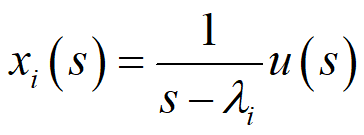其中：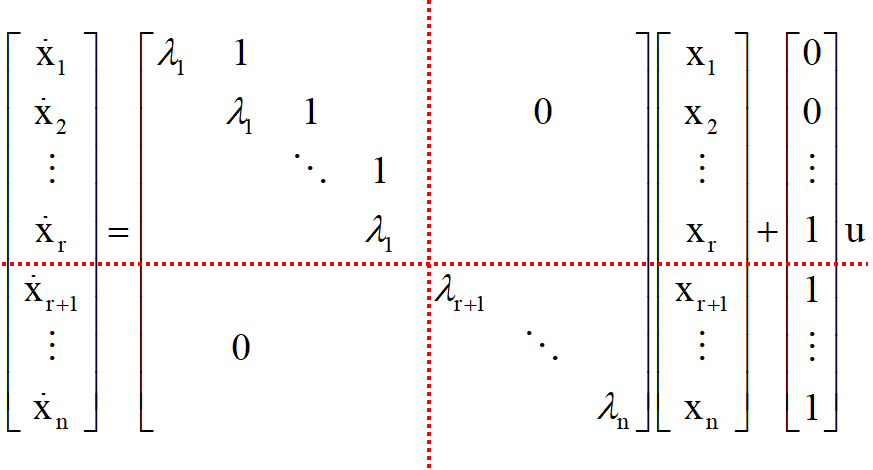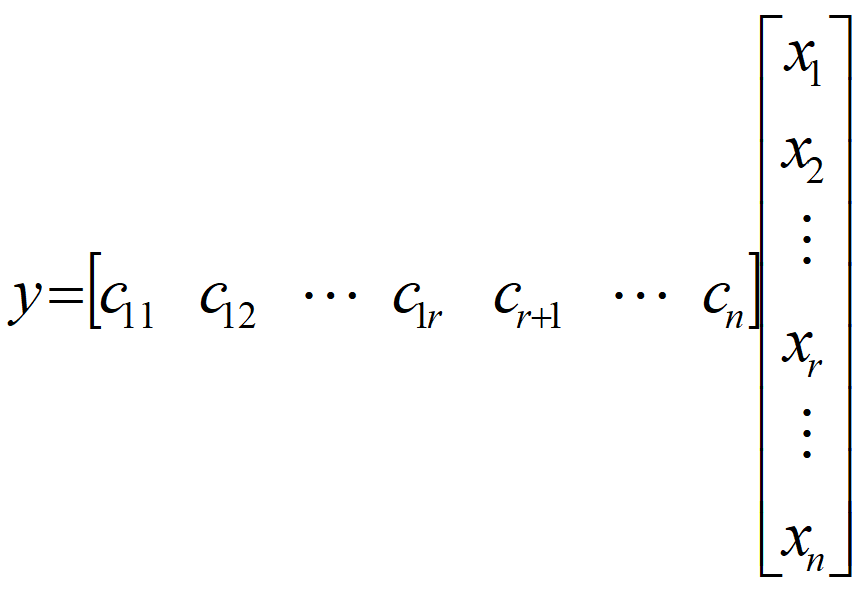展开全文• 介绍了从传递函数状态空间模型的基本方法、线性叠加法、串联法、并联法，从状态空间模型求传递函数的方法，传递函数状态空间模型的对偶性。


目录
一、传递函数

→

\rightarrow

状态空间模型1.1 预处理1.2 从最简单的分子为1的传递函数入手1.2 分子不为1的传递函数1.3 另一种思路：利用线性叠加原理1.4 对复杂系统使用分解法1.4.1 串联法1.4.2 并联法

二、状态空间模型

→

\rightarrow

传递函数三、对偶关系四、例题五、参考资料

传递函数是经典控制理论的工具，只能用于SISO和LTI系统；状态空间模型属于现代控制理论，对SISO和MIMO、LTI和非线性或时变系统都适用。既然考虑二者的互相转换，那么对象只可能是满足SISO和LTI的系统。

一、传递函数

→

\rightarrow

状态空间模型
传递函数的一般形式：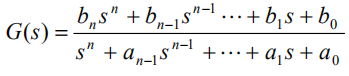1.1 预处理
用长除法进行简化：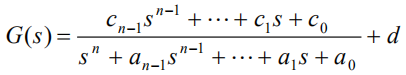从而得到新的G(s)：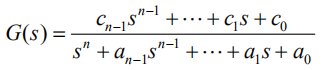原来的G(s)是新的G(s)和d的并联，可以按并联系统处理。方便起见，下面先只考虑形似新的G(s)的传递函数。
1.2 从最简单的分子为1的传递函数入手
一个例子：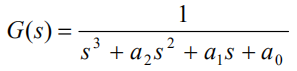要求它的状态空间模型，首先写成输入输出关系：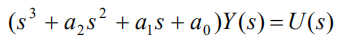对应的微分方程：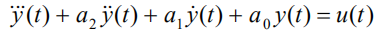定义新的变量：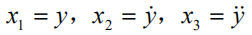状态空间模型：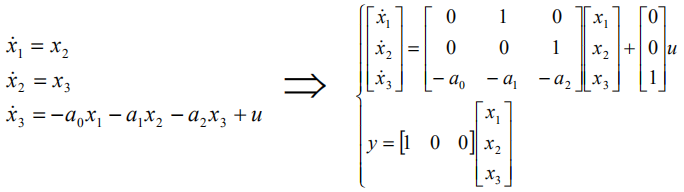状态矩阵A：最底行对应传递函数分母的系数，符号相反；右上角是2阶单位阵。 输入矩阵B：只有最后一维非零，对应分子常数1。 输出矩阵C：只有第一维非零，1对应分子常数1。 直接转移矩阵D：为0，因为G(s)的分子次数小于分母。 模拟图：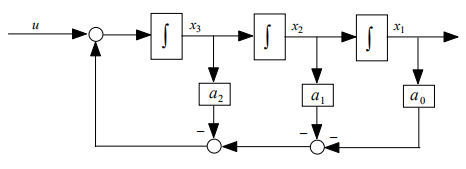画的时候左边是u，根据B中只有第三维是1，确定u通过加法流向

x

3

˙

\dot{x_3}

，积分得到

x

3

x_3

。然后再画

x

2

˙

,

x

2

,

x

1

˙

,

x

1

\dot{x_2},x_2,\dot{x_1},x_1

。根据

x

3

˙

\dot{x_3}

的构成画反馈。根据C得到

y

=

x

1

y=x_1

。
根据前面找到的规律，把上面的三阶传递函数，推广到一般情况：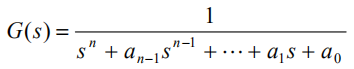对应的状态空间模型是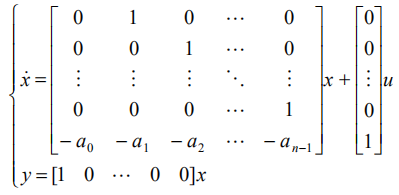称为是传递函数G的状态空间实现。
1.2 分子不为1的传递函数
还是考虑分母是三阶的：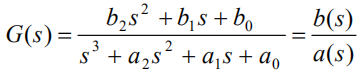可以看成是：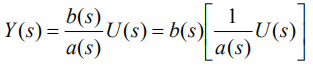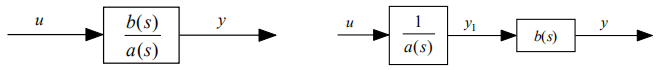对于内层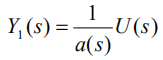的部分，显然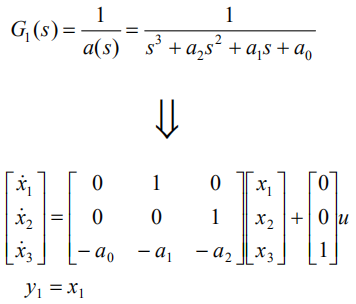对于外层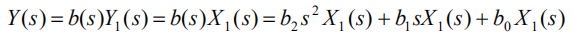微分方程：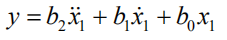定义状态变量：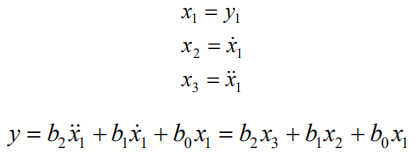这里的

y

1

y_1

也就是分子为1时候的y。也就是说，分子不为1的时候，输出是原来y及其微分的线性组合。所以，对于分子不为1的传函，状态空间模型是4个矩阵中只有B发生了变化：与传递函数的分子系数相对应。 相应的图为：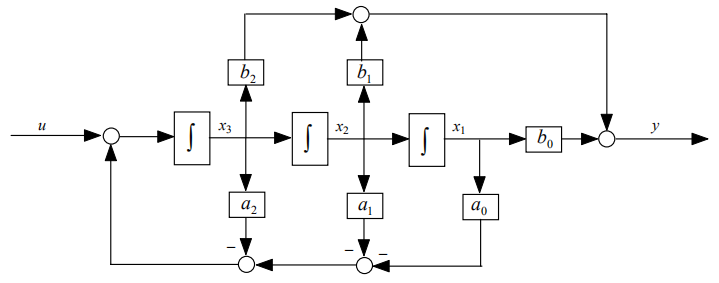画的时候，将3个积分器的输出线性相加得到最后的输出。
仍然把3维的推广到n维，并且考虑长除法得到的商：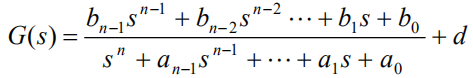对应的状态空间模型为：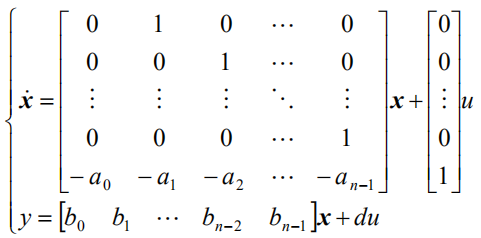只要把G化成上面的形式，就可以直接观察系数写出模型的4个矩阵了，很方便。这个形式的模型称为“能控标准型”。
1.3 另一种思路：利用线性叠加原理
还是以3阶系统为例：如果直接写成y和u的微分方程：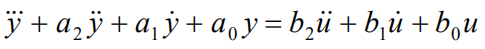这时候，右边的u是有微分项的（而按1.2中的方法，由于内层的分子是1，避免了这个问题）。 如果先不看微分项，只考虑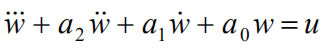它和前面分析过的分子为1的传递函数是一样的，即有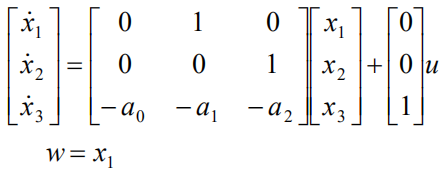然后只考虑u的一阶微分项作为输入：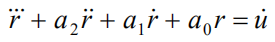对比上一个方程，可以发现这个方程是上一个方程两边再做一次微分的结果，所以输出分量r和w有这样的关系：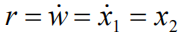同理，只考虑u的二阶微分的时候：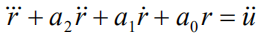又满足：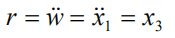根据叠加原理，总的输出是3个输入分量得到的3个输出分量的线性和：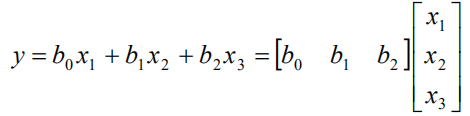b对应y-u微分方程右边的系数。
1.4 对复杂系统使用分解法
如果G的阶数比较高，可以把它分解成低阶的，先得到低阶的状态空间模型，再合成高阶的模型。可以用串联法、并联法等。
1.4.1 串联法
有的传递函数很容易因式分解，可以用串联法，例如：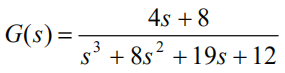因式分解：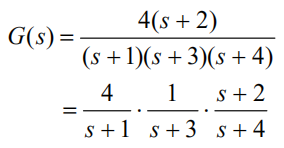看成是三个环节的串联，很容易得到它们各自的模型：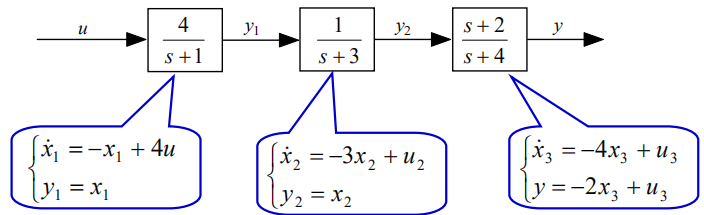系数的求法用之前的方法就可以做，前两个环节由于阶数很低，熟练之后可以直接看出来。对于第三个环节，我们可以设

X

3

(

s

)

=

1

s

+

4

U

3

(

s

)

X_3(s)=\frac{1}{s+4}U_3(s)

而

Y

(

s

)

=

(

s

+

2

)

X

3

(

s

)

Y(s)=(s+2)X_3(s)

，即可得：

x

˙

3

=

−

4

x

3

+

u

3

y

=

x

˙

3

+

2

x

3

=

−

4

x

3

+

u

3

+

2

x

3

=

−

2

x

3

+

u

3

\begin{aligned} \dot{x}_3&=-4x_3+u_3\\ y&=\dot{x}_3+2x_3\\ &=-4x_3+u_3+2x_3\\ &=-2x_3+u_3 \end{aligned}

这三个环节有什么关联呢？u2=y1,u3=y2。所以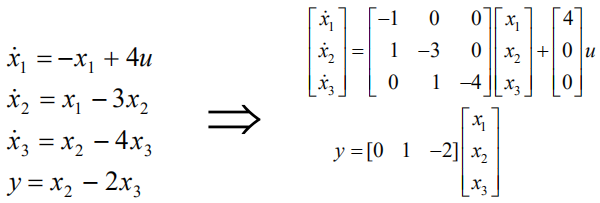图：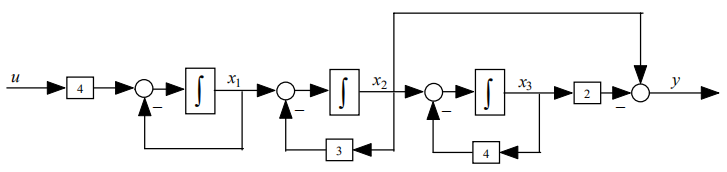1.4.2 并联法
考虑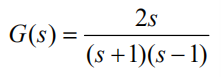分解得到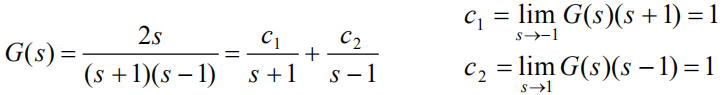画图，分别求出两个环节的模型：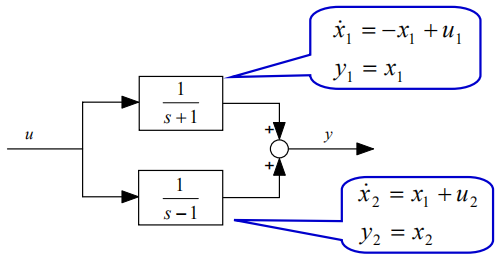对于并联，两个环节之间的关系是：u=u1=u2,y=y1+y2。因此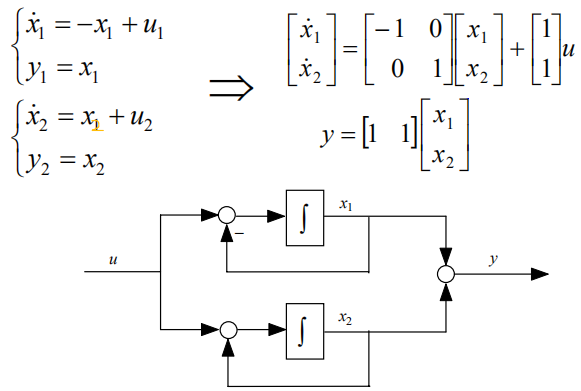这里的A是对角阵，所以并联得到的也称“对角型”。 系数的规律： A中的系数值是传递函数极点-1和1，B的系数对应分子1和1。 实际上，对于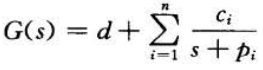利用并联法得到的模型为：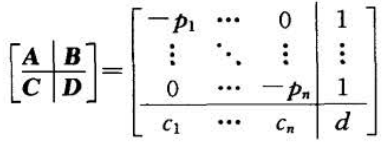状态图：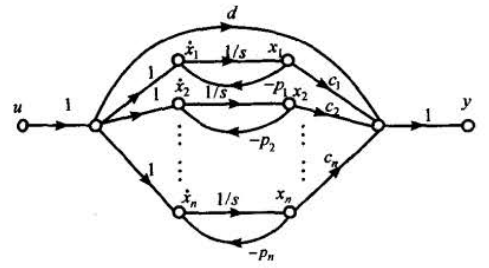如果存在重极点，例如：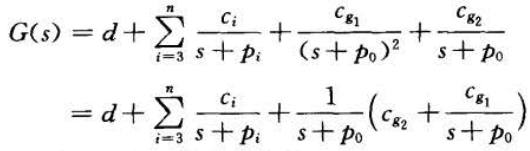系数用留数定理求：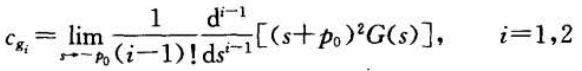输入输出关系为：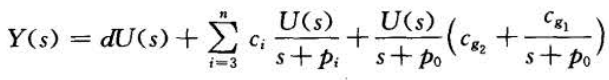令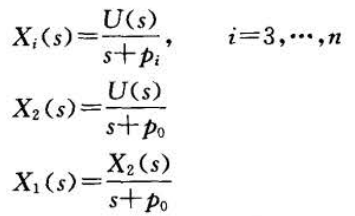得到系统状态方程和输出方程：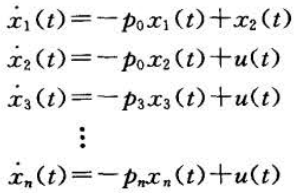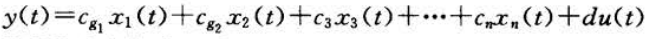因此，模型为：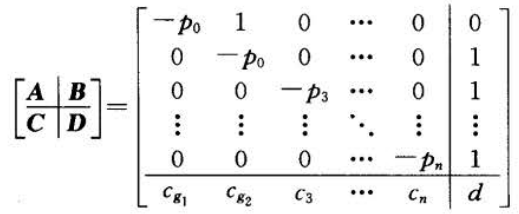观察发现，如果存在重根，则A为若当型，n重根对应的若当块是n阶的，并且相应的B中的系数为0。 状态图为：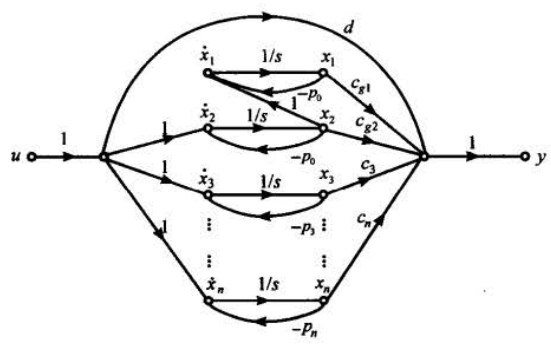二、状态空间模型

→

\rightarrow

传递函数
设已知的状态空间模型为：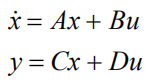在零初始条件下，用拉普拉斯变换得到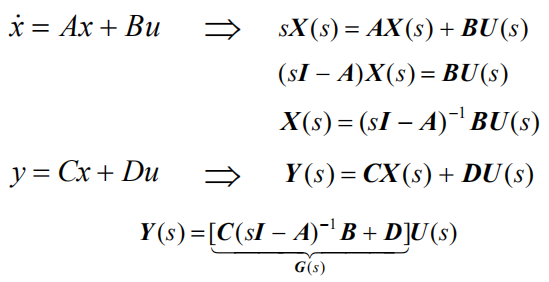所以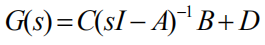可见，传递函数是由状态空间模型唯一确定的。
三、对偶关系
在2中根据状态空间模型可以求出传递函数：对于SISO系统，这是一个标量，所以，

G

=

G

T

G=G^T

。由此，可以得到：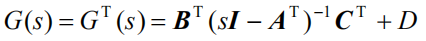这两种写法的形式是一样的，对比系数可以得出另一种模型写法：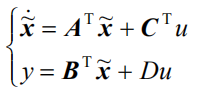这种写法的模型被称为原来模型的对偶系统模型。 对这个对偶模型再做一次对偶，可以得到原来的模型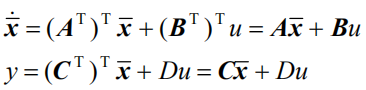这说明对偶关系是双向的，它们互为对偶。 我们上面写过能控标准型：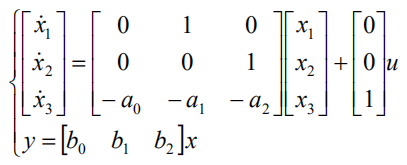它也有它的对偶模型，即“能观标准型”：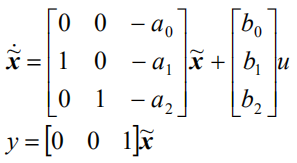两种模型的状态图：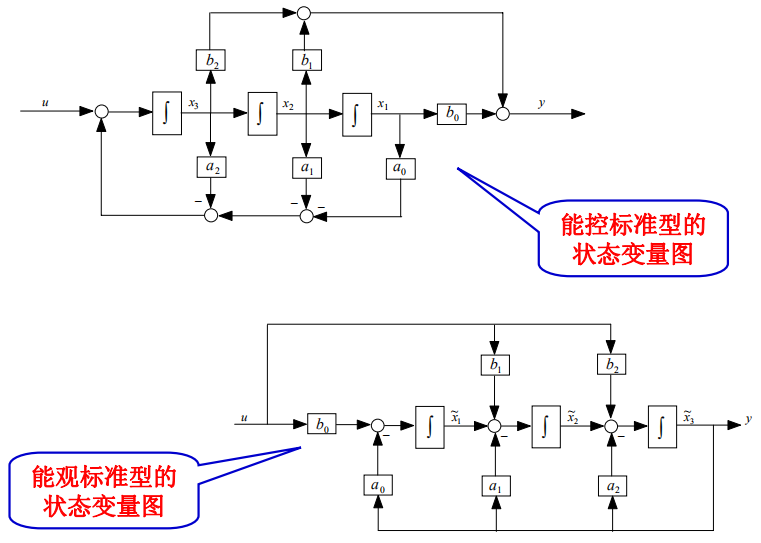四、例题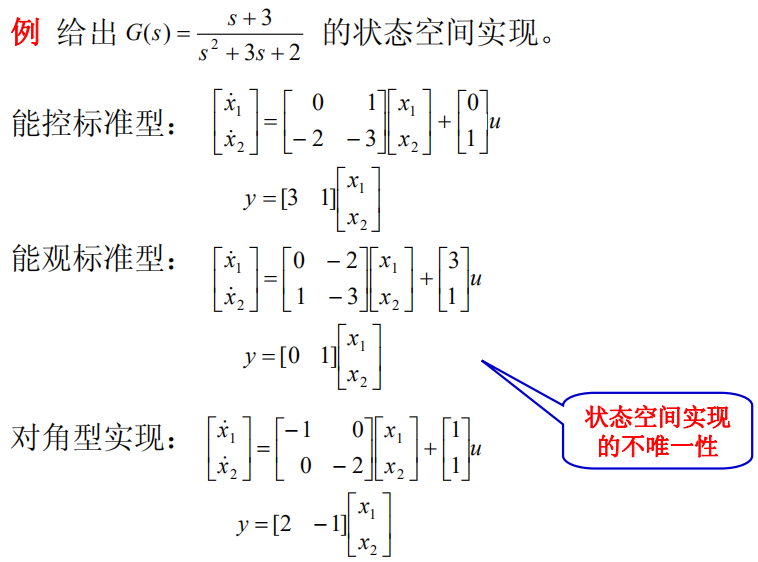五、参考资料
 浙江工业大学俞立老师课程ppt，网课见b站：现代控制理论 浙江工业大学 俞立，评论区有ppt的网盘链接  田玉平，蒋珉，李世华．自动控制原理[M]．北京：科学出版社，2006
展开全文自动控制原理
• 以下是PID控制的部分代码（matlab的m文件）：  ts=0.001;...把传递函数离散化（问题1）  [num,den]=tfdata(dsys,'v');离散化后提取分子、分母  rin=1.0;输入为阶跃信号  u_1=0.0; u_2=0.0; 什么


以下是PID控制的部分代码（matlab的m文件）：

ts=0.001;采样时间=0.001s

sys=tf(400,[1,50,0]);建立被控对象传递函数

dsys=c2d(sys,ts,'z');把传递函数离散化（问题1）

[num,den]=tfdata(dsys,'v');离散化后提取分子、分母

rin=1.0;输入为阶跃信号

u_1=0.0; u_2=0.0; 什么东西的初始状态（问题2）

y_1=0.0; y_2=0.0; 是不是输出的初始状态

error_1=0;初始误差

x=[0 0 0]';PID的3个参数Kp Ki Kd组成的数组

p=100;仿真时间100ms

for k=1:1:p

r(k)=rin;

u(k)=kpidi(1)*x(1)+kpidi(2)*x(2)+kpidi(3)*x(3)

if u(k)>=10

u(k)=10;

end

if u(k)<=-10

u(k)=-10;

end

yout(k)=-den(2)*y_1-den(3)*y_2+num(2)*u_1+num(3)*u_2;（问题3）

error(k)=r(k)-yout(k);

%返回pid参数

u_2=u_1;u_1=u(k);

y_2=y_1;y_1=yout(k);

x(1)=error(k);

x(2)=(error(k)-error_1)/ts;

x(3)=x(3)+error(k)*ts;

error_2=error_1;

error_1=error(k);

end

问题1：把传递函数离散化[SYSD,G]=C2D(SYSC,Ts,METHOD)这里面的method有好多种，而且用的method不一样得出的结果也不一样，这些参数究竟有什么区别？

问题2：这些是不是PID控制器输出的初始状态，“rin--①--PID控制器--②--被控对象--③---”是不是就是上面②的地方的信号值？

问题3（关键问题）：这个式子是怎么得出来的？从传递函数得出差分方程是个什么步骤，要具体点的或者给本参考书。

又如：在《先进PID控制MATLAB仿真（第二版）》P146有 被控对象G(s)=133/(s^2+25s)，采样时间为1ms，采用z变换进行离散化，经过z变换后的离散化对象为yout(k)＝-den(2)yout(k-1)—den(3)yout(k-2)十num(2)u(k-1)+num(3)u(k-2)（实在是搞不明白怎么来的）

问题4：不是线性的对象可不可以写成差分方程的形式，比如G(s)=20e^(-0.02s)/(1.6s^2+4.4s+1)带了个纯延迟的该怎么弄。或者换成个3阶对象怎么写成差分方程

最佳答案

1、c2d：假设在输入端有一个零阶保持器，把连续时间的状态空间模型转到离散时间状态空间模型。

[SYSD,G]=C2D(SYSC,Ts,METHOD)里面的method包括：

zoh 零阶保持， 假设控制输入在采样周期内为常值，为默认值。

foh 一阶保持器，假设控制输入在采样周期内为线性。 tustin 采用双线性逼近。

matched 采用SISO系统的零极点匹配法

2、只有U_1是2处的初始状态值，而U_2是用来传递U(k)的，所以U_2是U_1在下一个ts时间内的值

3、从差分方程获取传递函数：

y(k)+a1y(k-1)+……+any(k-n)=b0x(k)+b1x(k-1)+……+bmx(k-m)在零初始条件下对，对方程两边进行Z变换，得到该系统的脉冲传递函数G(Z)=Y(Z)/X(Z)=[b0z^m+b1z^(m-1)+……+bm]z^n/[z^n+a1z^(n-1)+……an]z^m 其中m《n

或等效形式G(Z)=Y(Z)/X(Z)=[b0+b1z^(-1)+……+bmz^(-m)]/[1+a1z^(-1)+……anz^(-n)] 其中m《n

从脉冲传递函数到差分方程

G(Z)=Y(Z)/X(Z)=[b0+b1z^(-1)+……+bmz^(-m)]/[1+a1z^(-1)+……anz^(-n)] 其中m《n,交叉相乘得Y(Z)[1+a1z^(-1)+……anz^(-n)]=X(Z)[b0+b1z^(-1)+……+bmz^(-m)]对X(z)和Y(z)进行z逆变换得到差分方程y(k)+a1y(k-1)+……+any(k-n)=b0x(k)+b1x(k-1)+……+bmx(k-m)

http://218.6.168.52/wlxt/ncourse/jsjkzjs/web/ppt/ch4.files/frame.htm

4、纯延迟系统G(s)=20e^(-0.02s)/(1.6s^2+4.4s+1)

num=;

den=[1.6 4.4 1];

sys=tf(num,den,'inputdelay',0.02)


展开全文• 原文地址：点击打开链接以下以双输入双输出系统为例:% Thansfer multiple transfer functions into sate space modelsclcclear all% Step1:输入传递函数分子分母系数%From u1 to y1num1=[-0.0006086 -7.715e-005];...
原文地址：点击打开链接
以下以双输入双输出系统为例:
% Thansfer multiple transfer functions into sate space models
clc
clear all
% Step1:输入传递函数分子分母系数
%From u1 to y1
num1=[-0.0006086 -7.715e-005];
den1=[1 1.079 0.2385 8.696e-005];
%From u2 to y1
num2=[0.03045 -0.0005537];
den2=[1 2.584 1.429 7.817e-005];
%From u1 to y2
num3=[3.135e-005 1.396e-007];
den3=[1 1.101 0.01934 5.657e-005];
%From u2 to y2
num4=[0.0003878 0.0001376];
den4=[1 0.6916 0.1158 0.003103];
% Step2:获得各单输入单输出状态空间模型
[A11,B11,C11,D11]=tf2ss(num1,den1);%From u1 to y1
[A21,B21,C21,D21]=tf2ss(num2,den2);%From u2 to y1
[A12,B12,C12,D12]=tf2ss(num3,den3);%From u1 to y2
[A22,B22,C22,D22]=tf2ss(num4,den4);%From u2 to y2
S_m11=ss(A11,B11,C11,D11);%From u1 to y1
S_m21=ss(A21,B21,C21,D21);%From u2 to y1
S_m12=ss(A12,B12,C12,D12);%From u1 to y2
S_m22=ss(A22,B22,C22,D22);%From u2 to y2
% Step3:按输入输出将状态空间模型写成矩阵形式,即为双输入双输出状态空间模型
P=[S_m11 S_m12;S_m21 S_m22];
% Step3:获得 A , B , C , D 矩阵
[A,B,C,D] = ssdata(P)

展开全文matlab
• 1 内容 有一个两输入两输出线性系统 ，求该系统的传递函数表达式子。...将状态空间表达式转换成传递函数的形式   参数含义 A, B, C, D代表状态空间表达式前面的矩阵，如在内容当中的状态空间表达式中，A代表矩阵
• 1.基本概念 状态方程：信号理论、控制理论与 热学都有状态方程，我现在指的是前者的。 状态：控制系统中 可决定系统状态的最小数目的一组变量...现代控制理论以状态空间理论为基础，经典控制理论以传递函数理论为基...
• Transfer function:  2 s^2 + 18 s + 40 ---------------------- s^3 + 6 s^2 + 11 s + 6   代码 num=[2 18 40]; den=[1 6 11 6]; %sys=tf(num,den);...实际上有传递函数转化成状态方程，状态方程的形matlab function c
• 最近一直在做毕设，期间也百度了很多网上的东西，也借了书来看，我所搜到的基本没有说的太详细的（可能是这个太基础了哈哈，大神都不屑于），那我就我所遇到的问题和方法... 1，已知传递函数，求出幅频特性。 ...matlab 幅频特性分析
• 今天想一个函数，从函数我需要的两个值传出来，由于传出来的值比较多，所以不考虑用return来返回，需要通过参数修改后的值拉出来供我使用，很当然的就想到了用指针，但是值就是传不出来；使我对原有的大脑中...c++ 指针
• 5.4.2状态空间模型函数 5.4.3 模型建立（MATLAB） 5.4 状态空间模型 5.4.1 概述 状态是系统动态信息的集合； 状态变量：在表征系统信息的所有变量中，能够全部描述系统运行/时域行为的最小数目的一组独立变量，...线性定常系统
• （2）将传递函数写成因式分解（零极点）形式 [z,p,k]=ss2zp(A,B,C,D) 或者 [z,p,k]=tf2zp(num,den) （3）将给定形式的状态空间方程变换成模态标准型 sysG=ss(A,B,C,D); [sysGm,TI]=canon(sysG,'modal'); [Am,Bm...matlab
• 编程中定义函数，一般三种传递方法，看是简单，想灵活合理选择，还需要大量的编程经验和技巧。 故在这里特意总结一下这三种传递的方法。 根本区别： 函数值传递不改变变量值，如果想改变变量值，需要返回值，然后...
• 之前在看北理工嵩天等老师的python3的课程，在第五周中老师讲到了函数的调用传递。老师讲了这样一个例子 #处理多个银行账户的余额信息 def addInterest(balances, rate): for i in range(len(balances)): ...
• ## 函数

千次阅读 2016-07-19 11:06:55
一、认识函数前面我们学习了程序设计中的三个基本控制结构（顺序、分支、循环），用它们可以组成任何程序，但在应用中，我们还要学习子程序结构。 通常在程序设计当中，我们会发现一些程序段在程序的不同地方反复...
• ## C++函数模板（模板函数）详解

万次阅读 多人点赞 2019-07-04 16:03:01
C++函数模板（模板函数...所有的类模板函数写在类的内部复数类：2.所有的类模板函数写在类的外部，在一个cpp中2.5总结关于类模板的几点说明：2.6类模板中的static关键字案例2：以下来自：C++类模板遇上static关键字...C++模板 类模板
• 状态空间方程的建立 基本概念： 状态：动力学系统的状态定义为信息的集合 状态变量：确定动力学系统状态的最小一组变量 状态向量：由n个状态变量组成的向量 状态空间：以状态变量位坐标构成的空间 状态方程：描述...经验分享
• ## Matlab常用函数

千次阅读 2014-06-03 10:15:00
Matlab有没有求矩阵行数/列数/维数的函数？ ndims(A)返回A的维数 size(A)返回A各个维的最大元素个数 length(A)返回max(size(A)) [m,n]=size(A)如果A是二维数组，返回行数和列数 nnz(A)返回A中非0元素的个数 ...
•c++ 深复制
• 你可以Callback函数看作是一种特殊的消息响应函数，一般来说我们不会自己调用这种函数， 而是有某些系统函数调用，而且不需要向后传递消息。 只要象C/C++这样支持函数指针的语言都 有回调函数的概念，它实际上是...查找文件
• ## C语言函数调用栈(一)

万次阅读 多人点赞 2018-07-19 22:16:25
编译器使用堆栈传递函数参数、保存返回地址、临时保存寄存器原有值(即函数调用的上下文)以备恢复以及存储本地局部变量。 不同处理器和编译器的堆栈布局、函数调用方法都可能不同，但堆栈的基本概念是一...寄存器 栈帧结构
• ## 函数式编程

千次阅读 2017-09-20 14:13:40
"函数式编程", 又称泛函编程, 是一种"编程范式"（programming paradigm），也就是如何编写程序的方法论。它的基础是 λ 演算（lambda calculus）。λ演算可以接受函数当作输入（参数）和输出（返回值）。lambda kotlin
• ## matlab函数总结

千次阅读 2018-07-28 10:38:58
ndims(A)返回A的维数 size(A)返回A各个维的最大元素个数 length(A)返回max(size(A)) [m,n]=size(A)如果A是二维数组，返回行数和列数 nnz(A)返回A中非0元素的...MATLAB的取整函数:fix(x), floor(x) :,ceil(x) , ro...matlab
• 文章目录 1. 基本概念 2. 系统的状态空间描述 状态空间描述框图 状态变量选取的非唯一性 3. 由系统微分方程列写状态空间表达式 一....相变量法 其他方法： 二....4. 由传递函数写状态...4. 由传递函数写状态空间表达式...
• ## Python 函数

千次阅读 2013-03-01 01:38:58
Python 第四部分 函数 目录 第15章 函数基础... 3 函数作用... 3 Def语句是实时执行的... 3 Python 中的多态... 4 第二个例子，寻找序列的交集... 4 什么时候python将会创建函数?. 5 检查传入函数的...python
• 文章出处：...   1. 同步函数与异步函数  什么是同步函数？  什么是异步函数？  它们在线程中执行时会对线程有何影响？  一个线程如何与一个异步执行的函数进行同步？ 依据微软的MSDN上的解说windows 多线程 winapi api
• 利用好函数能够充分利用系统库的功能出模块独立、易于维护和修改的程序。函数并不是 C 语言独有的概念，其他语言中的方法、过程等本质上都是函数。可见函数在教学中的重要意义。在教学中一般采用画简单的堆栈图的...
• ## 中断函数

千次阅读 2014-10-22 12:44:15
C51的中断函数的格式为：void FuncIr(void) interrupt x [using y] 以下是梦游的一些分析：  一、中断函数是一个特殊的函数，没有参数，也没有返回值；但是程序中允不允许使用return呢？答案是允许的，不过只能用......